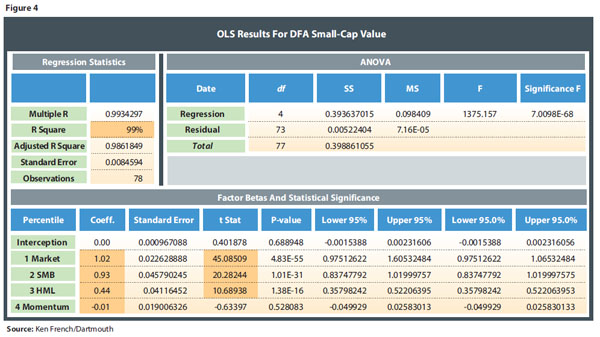# Cloning DFA

January 28, 2015

From there, calculate the Fama-French factors in the usual way—ordinary least squares (OLS) regressions. The DFA returns are the dependent variable; the “market return,” “small cap” (SmB), “value” (HmL) and “momentum” premiums are the independent variables (Figure 4).We added a factor for “momentum” because DFA started using momentum around 2005. When we add momentum, the model technically is referred to as the Carhart four-factor model. The R-squared in Figure 4 tells us that the model can explain 99 percent of DFA’s return variability. That’s an impressive number that tells us that the factors for market beta, small-cap effect (SmB) and value effect (HmL) are each very strong when explaining DFA’s returns. The betas may look small, because numbers like 0.44 and 0.93 are tiny, but don’t let that fool you. The t-stat is the true measure of how statistically significant the beta coefficients are and the relative strength of a prediction; it’s technically more reliable than the regression coefficient because it takes error into account.

As you may recall from sophomore statistics, the greater the t-stat, the greater the relative influence of the independent variable on the dependent variable. A t-stat over 1.96 is considered strong, so the t-stats we see in Figure 4 of 45, 20 and 10 are huge. Simply put, it means the generalizability of the findings beyond the sample is safe. In our case, the “market,” “small-cap” and “value” factors are absolutely, unequivocally driving DFA’s returns. The intercept is what industry practitioners would refer to as “alpha.” but the coefficient is small (0.00) and the t-stat insignificant. This implies that DFA does not supply any additional value after taking into account the “small-cap” and “value” factor loads.

Next, you have to address how you want to replicate the Fama-French factors. It doesn’t make sense to try and match all the betas at once. From a data perspective, we have to take into account statistical significance as well as relevance. The “market” beta has the largest t-stat—over 45, which is enormous, so it’s the most significant factor. A few points off on that beta are far more important than a few points off on “size,” “value” or “momentum.” The “small-cap” effect (SmB) is the next most important, and then “value” (HmL). Only factors that are statistically significant need to be targeted; theoretically, you’d like to keep the betas in balance proportional to each factor’s t-stat and p-value.

We require that the differential error in SmB betas be smaller than the differential error in HmL betas because SmB is more statistically significant, but we have to be cognizant that a numerical difference in betas is more meaningful when the numbers themselves are smaller. We have to scale the differences in the size of the coefficient betas as well as take into account the volatility of the coefficient. If a factor has a large expected return associated with a very small beta, then we need to make sure the clone doesn’t stray too far from that low, but powerful, beta.